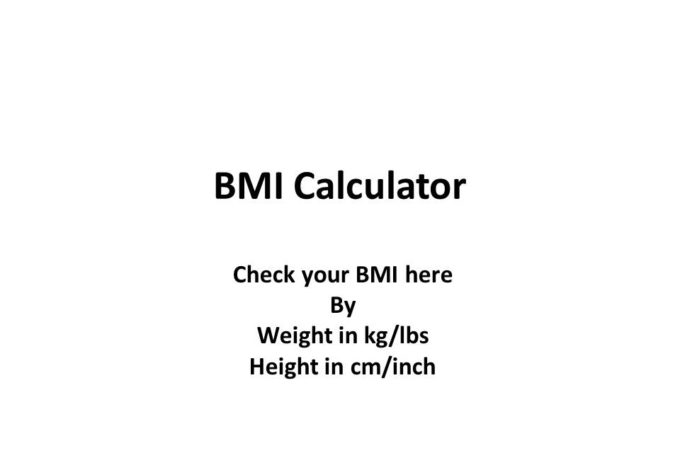# BMI calculatorBMI means Body Mass Index

### BMI calculator

ft
in
lbs
cm
kg
 (Body Mass Index) Range Status Below 18.5 Underweight 18.5 -24.9 Normal 25 – 29.9 Overweight 30 & Above Obese

## Formula

Body Mass Index = Weight[kg] / ( Height[m] x Height[m] ) = 703 x Weight[lb] / ( Height[in] x Height[in] )

kg-weight in Kilogram

m-length in meter

lb-weight in pound

in– length in inch

 Body Mass Index for Male and Female Men Women Status < 20.7 < 19.1 Underweight 20.7 – 26.4 19.1 – 25.8 Normal 26.4 – 27.8 25.8 – 27.3 Marginally overweight 27.8 – 31.1 27.3 – 32.3 Overweight > 31.1 > 32.3 Obese